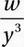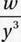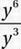# SAT Math Multiple Choice Question 715: Answer and Explanation

### Test Information

Question: 715

10. If y = x2, where x ≠ 0, and w = y6, which of the following expresses the value ofin terms of x?

• A. x2
• B. x4
• C. x5
• D. x6

Explanation:

D

Algebra (exponentials) MEDIUM-HARD

Expression to be evaluated:Substitute w = y6:Simplify with Exponential Law #6 (from Chapter 9, Lesson 9):

y3

Substitute y = x2:

(x2)3

Simplify with Exponential Law #8 (from Chapter 9, Lesson 9):

x6